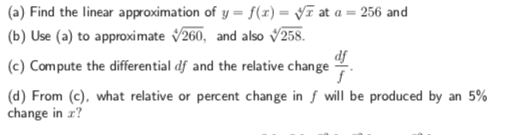# (a) Find the linear approximation of y = f(z) = yr at a = 256 and (b) Use (a) to approximate 260, and also v258. (c) Compute the differential df and the relative change (d) From, (e), what relative or percent change in f will be produced by an 5% df change in r?

Questionhelp_outlineImage Transcriptionclose(a) Find the linear approximation of y = f(z) = yr at a = 256 and (b) Use (a) to approximate 260, and also v258. (c) Compute the differential df and the relative change (d) From, (e), what relative or percent change in f will be produced by an 5% df change in r? fullscreen

### Want to see this answer and more?

Experts are waiting 24/7 to provide step-by-step solutions in as fast as 30 minutes!*

*Response times vary by subject and question complexity. Median response time is 34 minutes and may be longer for new subjects.
Tagged in
MathCalculus

### Derivative